# Using Annotation Plots in Your 2D and 3D Plot Groups

February 3, 2016

Adding annotation plots to your plot groups is an easy way to label the plots of your simulation results with names, comments, and values of quantities evaluated at specified locations. In this blog post, we’ll explore adding annotation plots to a model of a heat sink.

### What Are Annotation Plots?

Annotation plots, new with COMSOL Multiphysics version 5.2, can be added to 2D and 3D plot groups to create visible notes or comments in your model results. They appear within a Plot Group node in the same way as they do for any other plot: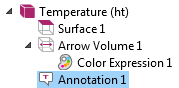The Settings window allows you to change the annotation’s details, including the position of the comment in the coordinate system of the model, the text in the comment, the degree of precision in the values displayed, certain style settings such as font color, and the title of the plot: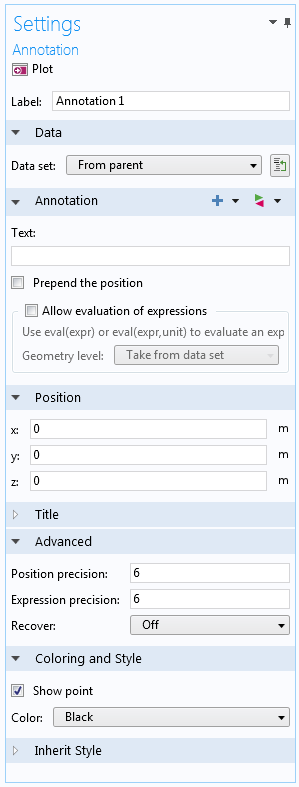The settings for an annotation plot.

I’ll walk through some of these settings in the heat sink model to demonstrate how the process works.

### How to Add Annotations in 2D and 3D Plots

Any time you’re working in a 2D or 3D plot group, you can right-click on the Plot Group node and choose More Plots > Annotation to add an annotation plot. In the heat sink simulation, I added an annotation plot to the Temperature (ht) plot group that is available in the solved model: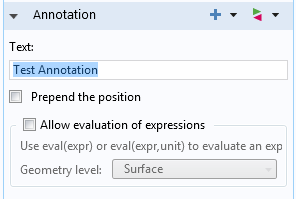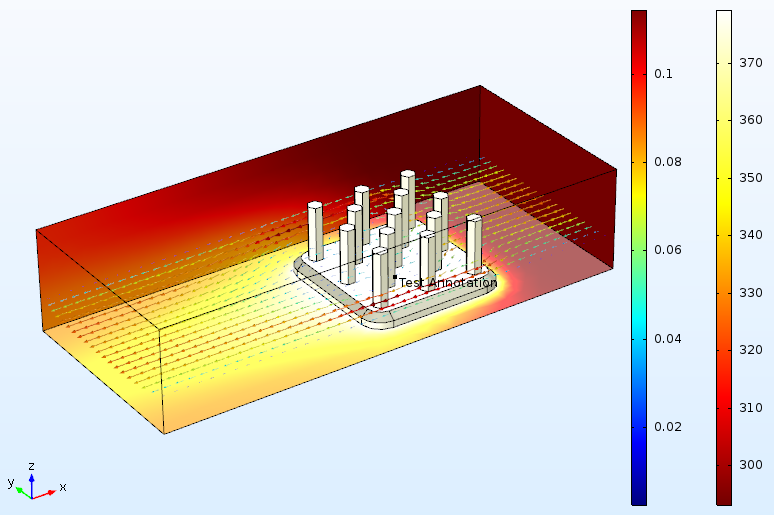By default, the annotation is positioned at the coordinates (0,0,0). Above, the note appears overlapped with the other plots in the plot group.

One very simple use of annotation plots is to use them as labels. In the following graphic, I have added three annotation plots to the heat sink results in order to indicate different parts of the geometry and the meaning of the arrow volume plot: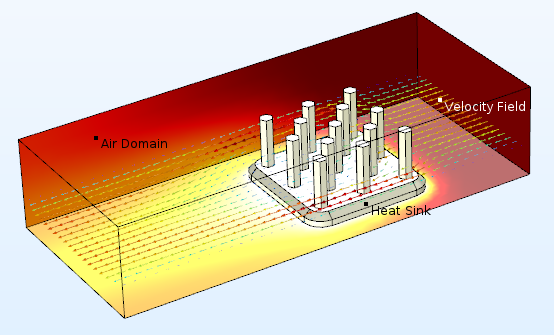For a 2D plot group, the annotation settings function similarly, except for the position settings, which have been reduced to x– and y-coordinates. The following plot shows an annotation in a 2D plot group where the temperature is displayed on a cut plane running down the center of the heat sink: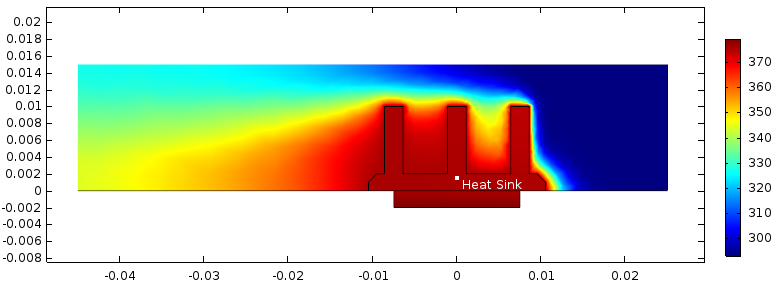### Evaluation of Expressions in Annotation Plots

A more common and useful way to use annotation plots is to include the value of a chosen expression at a particular point in the model geometry. With the Allow evaluation of expressions check box, the annotation is permitted to display the evaluation of a specified quantity. In the image below, I’ve chosen to evaluate the temperature in the heat sink at the coordinates of my annotation.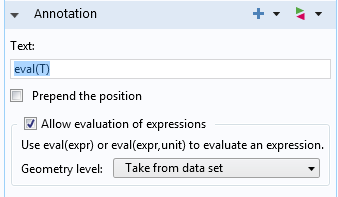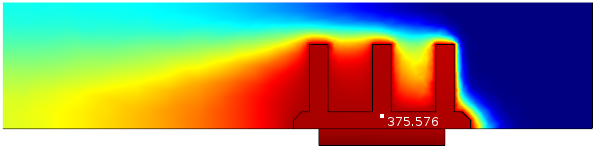It’s also possible to plot on entities of a lower dimension than the plot group: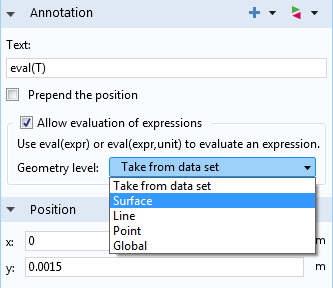For annotations created in a 3D plot group, plotting on volumes is also an option.

Allowing the evaluation of plot expressions in an annotation means that the position of the label dictates the results. For instance, if I shift the position of my annotation plot to (0.01, 0.01), I will see different temperature results than I did at (0, 0.0015) in the previous plot: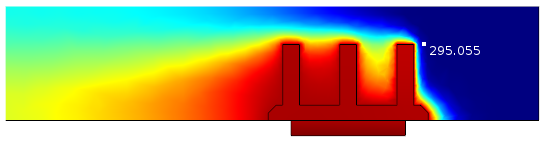The Prepend the position check box can be especially helpful when visualizing the value of a quantity at a certain location. Checking this box will add the coordinates of the annotation before the text: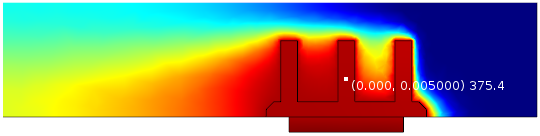The eval(expression) command is flexible — these methods can also be applied to time-dependent studies, for instance. The Replace Expression menu is accessible from the Annotation section of the settings and gives access to the same plot expressions that are available in other plot types.

Stay tuned for more postprocessing discussions on the COMSOL Blog.

### Explore Other Postprocessing Resources

#### Comments (0)

##### Leave a Comment
Log In | Registration
Loading...
EXPLORE COMSOL BLOG